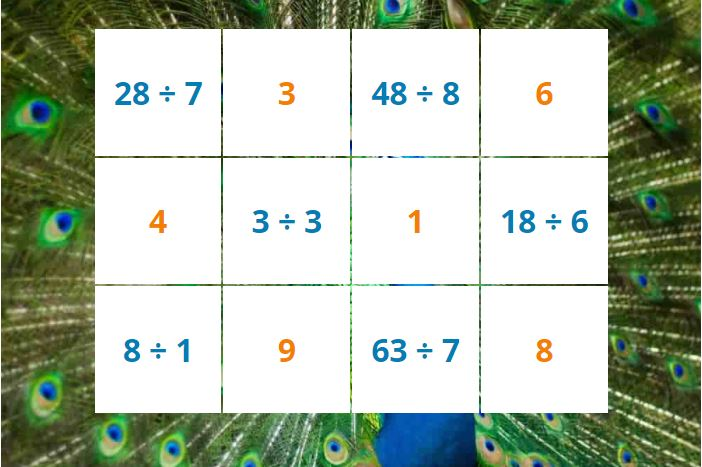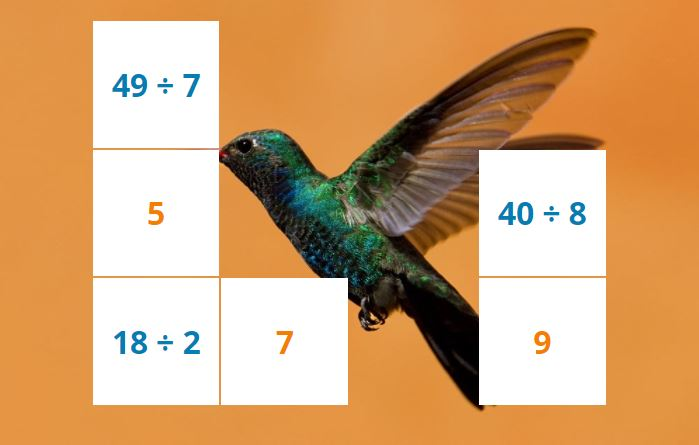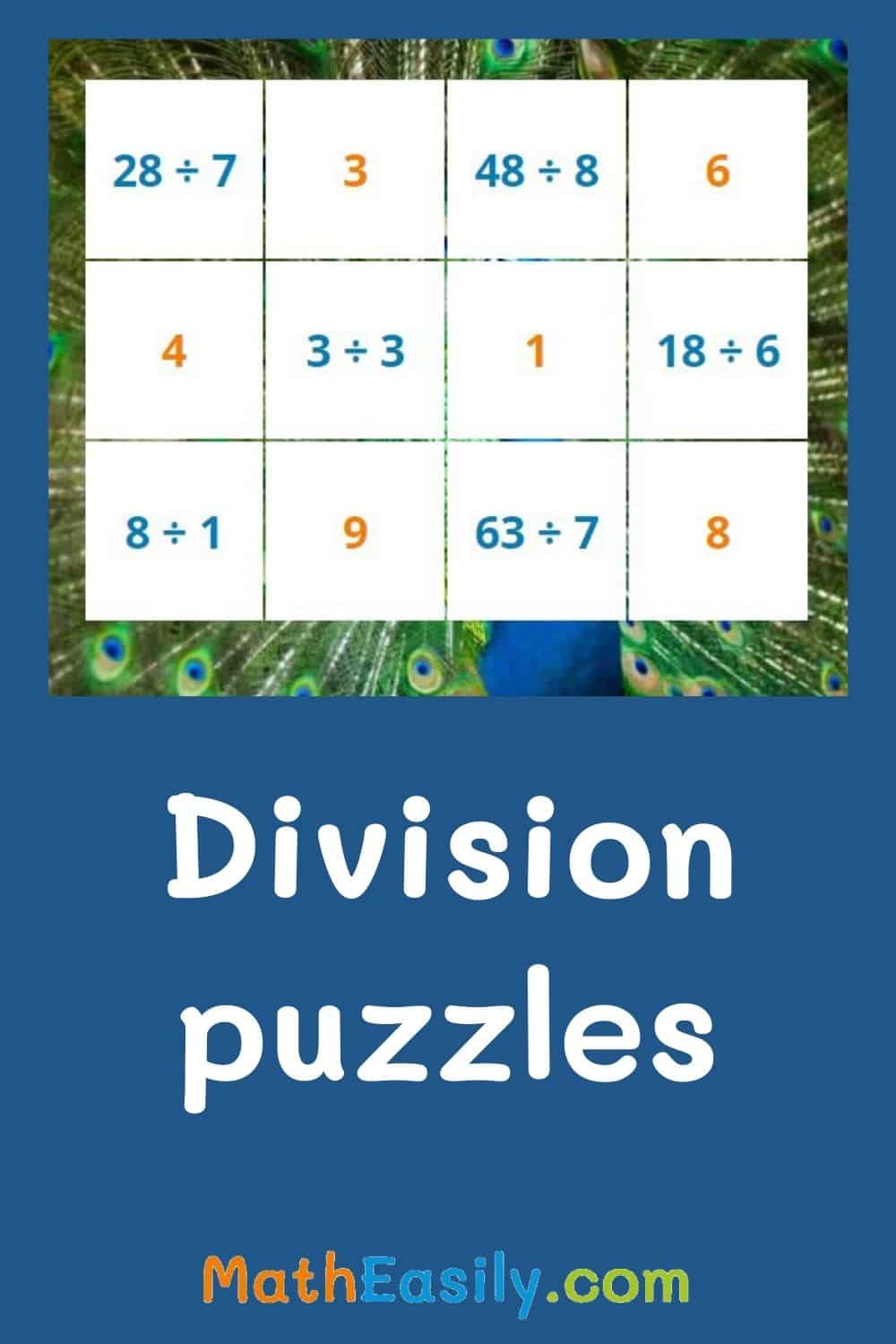﻿ Math division puzzles ONLINE + Printable

Page No. 1025

# Division puzzles

Play math division puzzles for grade 2. Download printable division puzzle worksheets. Multiplication and division puzzles 3rd grade. division pictures. Puzzle pics division. math division puzzles for grade 3. Division sqaure puzzle. math division puzzles for grade 4. division picture puzzles. Division puzzle game. Printable division puzzles PDF. math puzzles division.

Solve division puzzles online: click on 2 tiles so that they contain the same result.
Watch as the animal picture appears.

## Math division puzzlesHalf of the tiles contain the division problem (blue text) and the other half contain the result (orange text). Students have to click first on the task and then on the corresponding result. They are looking for pairs of division puzzles: the division task and the result.

When the children manage to find a pair of tiles that belong together, the tiles disappear. When kids click on 2 tiles which don´t match, the tiles will flash red.

Did you click on a tile by mistake? It doesn't matter, click it again, the tile will be white again.

Continue to play division puzzles until all tiles disappear. Then press the button "New puzzle" and a new online division puzzle will be generated.To play division puzzles kids have to have a basic knowledge of division. To practice division tasks try these online division flash cards.

## Printable division puzzle worksheets

These online division puzzles complement with printable division puzzle worksheets. Printable division picture puzzle are designed for an use in the classroom or for home schooling. Everytime 12 new different division problems are generated and create a new image. More than 50 pictures available.

## Puzzle pics division

Math division puzzles are a great method how children learn division in a fun way. As kids complete the division tasks, they gradually uncover pictures of animals.

They look forward to what they see in the picture and therefore find the division problems easy. Download division picture puzzle below or browse all our free division worksheets here.

Do you like division puzzles?
Then try also our multiplication puzzles.## Division puzzles ONLINE

Math division puzzles are one of our most popular online division games. Learn to divide easily with these division picture puzzles.

These division puzzles are designed in a way that allows children to work at their own level. The child can start with the easiest division tasks and continue towards more difficult division problems.

## Division picture puzzles

Division picture puzzle takes advantage of children's curiosity. Children love to solve easy division problems to complete the division square puzzle. You can find more similar printable math puzzles here.

### Math division puzzles

Division puzzles are mathematical puzzles that involve dividing numbers to find a solution. In these puzzles, the quotient is missing, and you need to find its value. For example, you are given an equation like "24 ÷ 8 = ?" The task is to determine the missing quotient by dividing the dividend (24) by the divisor (8) by choosing from 6 options.

Because division can be an abstract concept for young kids, you can explain division as a way of sharing or grouping things equally. This game Division with pictures is super illustrative for this purpose.